# Secure Transfer of Color Images Using Horizontal and Vertical Scan

Secure Transfer of Color Images Using Horizontal and Vertical Scan
LASA Laboratory, Badji Mokhtar University, Annaba, Algeria

Electronics Research Laboratory, University of 20 August 1955, Skikda, Algeria

Corresponding Author Email:
oulayab@yahoo.com
Page:
45-51
|
DOI:
https://doi.org/10.18280/ts.360106
5 November 2018
|
Accepted:
13 January 2019
|
Published:
30 April 2019
| Citation

OPEN ACCESS

Abstract:

The aim of this contribution is to enhance the security of encrypted image and decrease the execution time. The proposed cryptosystem based on the keystream generator (KSG) inspired from achterbahn-128 characterized by some good properties used for generating the key. The scan pattern is utilized for the mapping of the image encryption. After the generation of the keystream, the image is encrypted vertically using the scan pattern partition, and then the encrypted image is permuted and rescanned horizontally. Several tests are done using MATLAB software in order to prove performances of the system, including the statistical tests, the sensitivity analysis, and the security analysis. The proposed technique is simple to implement and has high encryption rate.

Keywords:

image, encryption, decryption, scan pattern, stream cipher, keystream generator, permutation, NLFSR

1. Introduction

With an astounding growth in the field of multimedia and network technologies, the security of multimedia data becomes much important in data storage and transmission. Thus the information that circulates over unsecured channels is a subject to several types of attacks (interception, modification of the content ... etc.) carried out by pirates (cybercriminals), the latter keep improving their techniques and their methods [1-5]. Images are a part of multimedia and hugely used in various applications, like multimedia systems, internet communication, military image databases and medical imaging system, etc.

Image encryption is more complicate than text encryption. The specific characteristics of this type of data  (high redundancy, high bulk capacity, strong correlations among adjacent pixels, not sensitive as text) make standard encryption algorithms inadequate.

Generally the stream cipher is based on LFSR (Linear Feedback Shift Register), NLFSR (Non Linear Shift Register), and combined function. They are widely used in secure communication like: mobile communication and Bluetooth, because of their high throughput, less complex hardware circuitry and very little error propagation which has attracted much attention. Improved Achterbahn-128 key stream generator  is a bit oriented synchronous stream cipher based on the combination of seventeen NLFSR (Non Linear Feedback Shift Register) of Boolean function with good properties that ensure an adequate security. But these systems are unsuitable with image encryption due to the length of the key which has the same size as the image to be encrypted. This task involves a long processing time and high computing power.

On the other hand, the SCAN pattern is a block cipher like AES (Advanced Encryption Standard), DES (Data Encryption Standard) or IDEA algorithms that can be used to encrypt the transmitted data over a network. The main advantage of SCAN methodology for image encryption and decryption is the high throughput and a good security that can be increased using several encryption loops.

In this paper we are interested in building a new fast scheme for colored images encryption and decryption that provides a maximum security combined between the scan pattern for double scanning of the image and the improved Achterbahn-128 keystream generator for key generation.

The paper is organized as follows. The section two gives a brief description of the scan pattern and description of the existing methods. In section three the proposed method is given in detail. In section four we present an implantation of the proposed scheme for image encryption and we give the security analysis of the design. Finally, the section five concludes the paper.

2. Existing Methods

The scan pattern as defined by Maniccam and Bourbakis  is a formal language-based two dimensional spatial accessing methodology which can represent and generate a large number of wide varieties of scanning paths. The scan language uses four basic scan patterns such as continuous raster (C), continuous diagonal (D), continuous orthogonal (O), and spiral (S). The basic scan patterns are shown in Figure 1.

## 1.png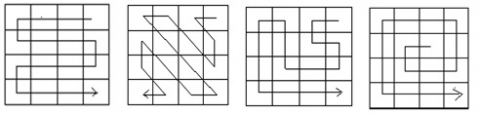Figure 1. Graphical representation of the SCAN patterns

The basic SCAN language is composed by three basic partitions: (B); (Z); (X) partition patterns.

Each of which has eight transformations numbered 0 to 7 . The trans-formations 1, 3, 5, 7 are reverse of transformations 0, 2, 4, 6, respectively as shown in Figure 2.

## 2.png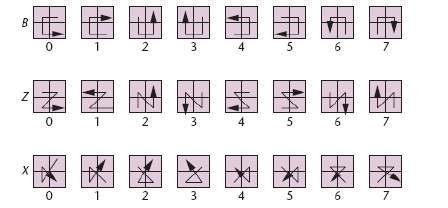Figure 2. The different partitions of scan patterns

The main advantage of the SCAN algorithm is its strong encryption rather than its high throughput . The properties of the SCAN image encryption method are the inclusion of pixel rearrangement, confusion, and diffusion. The Simple Scan Pattern (SSP) is an algorithm that calculates the scan address using some iterative loops, the software implementation of the scan algorithm consists of two nested loops.

Since 1999, some methods based on the scan patterns for image and video encryption have been proposed in literature [11-16] the scan pattern was used to design a scan transposition cipher (STC) and the combination with some methods as quadtree decomposition and the 2DRE (2Dimensional Run Encoding) to encrypt images. These methods were insecure against known plaintext and chosen plaintext attacks [17-19].

In 2006, Chaos Shen Chen in  proposed an image encryption and decryption process by rearrangement of the pixels of the image. Rearrangement was performed by SCAN patterns that generated by scan methodology.

Panduranga and Naveen Kumar  proposed a hybrid technique for image encryption which employs the concept of carrier image and the SCAN patterns that are generated by SCAN methodology, the novelty of the work lies in hybridizing and carrier image creation for encryption.

In 2012, Nagaraju et al.  proposed a new image encryption method using secret-key images and SCAN patterns. After creating a key image, it is added with the original and then the SCAN pattern is applied, this is generated by the SCAN methodology at the original image or key image.

Sivakumar and Venkatesan (2016) proposed a novel image encryption method using scan pattern, circular shift, and transposition methods . The pixels of the original image are permuted by the scan pattern that is generated by the notion of Kth smallest and stacked dynamically. The circular shift and transposition are done by Shuffling Key (SK) generated from the original image; the obtained algorithm is fast and weak against the statistical attacks.

3. Method Desription

The inherent disadvantage in the stream cipher is the length of the key which is the same size as the message to be encrypted. This disadvantage makes its systems unsuitable for the encryption and decryption of big data despite the sufficient security that provides. This work meets these requirements with the minimization of the length of the key as much as possible with a reinforcement of security. This work is hybridization between the bloc cipher and stream cipher. The Figure 3 shows the bloc diagram of the proposed method.

After loading the key stream by the key bit generator (PRG), firstly, the image is encrypted vertically by the vertical scan generated by the scan patterns with Xor bit per bit then the lines are permuted in order to not have decryption of the first pixels of the image. Secondly, the obtained image is re-encrypted by horizontal scan that is generated by the scan patterns; in the decryption process the reverse functions are preceded.

## 3.png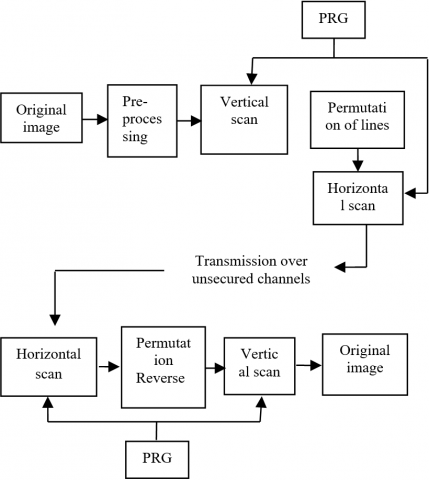Figure 3. Bloc diagram of the proposed method

3.1 The vertical and horizontal scan

In this paper, we have used a double scan for reinforcing the security, at the same time we have decreased the length of the key stream, which is generated by the generator described in the section 3.3. Two sorts of scan are used namely: the vertical and horizontal scan. In the vertical scan we have used the partition pattern Z(6) and in the horizontal scan we have used the partition pattern Z(0) as shown in Figure 4.

## 4.png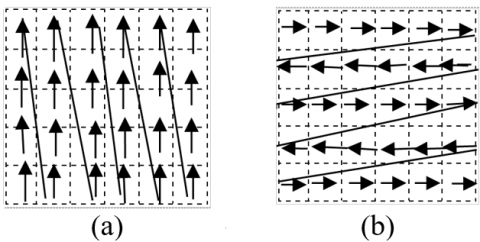Figure 4. The proposed scan scheme: (a) the vertical scan, (b) the horizontal scan

3.2 The permutation process

The permutation operation changes only the pixel positions but not the pixel values for the plain-image. Generally, the permutation techniques work as follow: the image can be decomposed into sub blocs and each one contains a specific number of pixels, the sub blocs are transformed into a new location. In this paper, after the vertical encryption, we are used a permutation of lines in order to not have decryption of the first pixels of the image in the second encryption.

3.3 Key stream generator

In order to implement the proposed approach for image encryption and decryption, we propose to use the key stream generator presented in . The generator is a stream cipher system that consists of seventeen non linear feedback shift registers (NLFSR) denoted $R_j$ of lengths $l_j$,where $1≤j≤17$, and a non linear combining function Hof 17 variables.

The NLFSRs are such that they can produce binary sequences of period $2^{l_j-1}$. The nonzero output sequences of the seventeen binary NLFSRs are taken as input to the combining function H. The key stream sequence (zi)i≥0 is computed as

$z(i)=H(x_1 (i),x_2 (i),…,x_17 (i)),∀i≥0$ (1)

where $(x_j(i))_i≥0$ denotes the output sequence generated by the j-th element of the NLFSR and H is a function of 17 variables.

The variables

$x_1 (i),x_2 (i),x_3 (i),x_4 (i),x_5 (i),x_6 (i),x_7 (i),x_8 (i),x_9 (i),x_10 (i),x_11 (i),x_12 (i),x_13 (i),x_14 (i),x_15 (i),x_16 (i)$ and $x_17 (i)$ corresponds to the tap positions 19, 39, 34, 23, 25, 36, 26, 29, 27, 28, 30, 40, 31, 44, 45, 32 and 33 respectively.

Each shift register is described by its feedback function. The algebraic normal forms of the feedback functions are given by .

The combining function H is balanced, number of variables is 17; it has an algebraic degree of 7; the correlation immune is of order 9; H has maximum nonlinearity of 64512. The algebraic normal form of the Boolean function is given by .

## 5.pngFigure 5. Nonlinear combination generator

3.4 Encryption and decryption algorithm

In this method, we have used two kinds of scan method for selecting the mapping of the image encryption in order to decrease the length of the key stream, Instead of generating a sequence of size (L *M*3), we generate a key sequence of size L or M according to the largest, by the stream cipher improved Achterbahn-128 generator. In the decryption process we always ensure that the information is received by the receiver, the inverse operation is applied to the encrypted image in order to obtain the original image.

At the input of the system, the plain image digit x of size L×M×3 and the initialization of the seventeen NLFSR which is the secret key. The algorithm illustrates the key stream generation process.

3.4.1 The key generation

Inputs:

x: plain image digit;

l0,l1, …, l16: the NLFSR are initially loaded by the secret key;

H: combining function with 17 variables.

Outputs:

sj: the sequence produced by the NLFSRs, z: Key stream produced by H

Treatment:

1. to read M, L,P respectively the length of row, column, and the color of the plain image digit x;
2. Introduce the secret key, the value of Initialization of 17 NLFSRs;
3. Calculate N=MAX (L, M)
4. For i=1 to (N*8)to make;
5. Generate the binary sequences sj(i) produced by 17NLFSRs ;
6. End to make;
7. For i=1 to N to make;
8. Generate the key stream z(i) produced by function H;
9. End to make.

3.4.2 The encryption process

The encryption process is divided into three phases, each plan color is encrypted separately, at the first the image is encrypted vertically column per column by scan partition pattern (Z6) as shown in Figure 2, after that the lines are permuted. The flow chart illustrates the encryption process of the proposed method.

3.4.3 The decryption process

## 6.png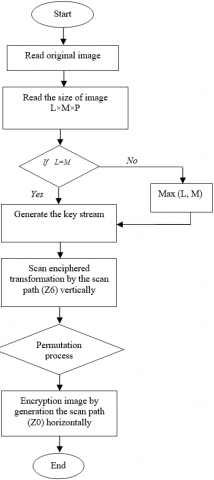Figure 6. Flow Chart of encryption process

The decryption process involves the reconstruction of the original image by following inverse steps. After the reception of the encrypted image, the image is decrypted horizontally by the scan path (Z0) then the permutation inverse of lines is applied. Finally, the image is decrypted vertically by the scan path (Z6) in order to obtain the original image (See Figure 6).

4. Results and Discussion

To validate the proposed approach carried by Matlab v7.10a several tests are done to grayscale and colored images with deferent sizes. In this paper we have taken as example the colored image peppers.png of size (512*512*3) pixels.

From the original image shown in Figure 7, we applied the encryption process described in section 3.4.b in order to obtain the encrypted images shown in Figure 7. By comparing the original image and the encrypted image, there is no visual information observed in the encrypted image with a big difference found in the original image.

## 7.pngFigure 7. Typical results of the encryption process of the proposed system

## 8.png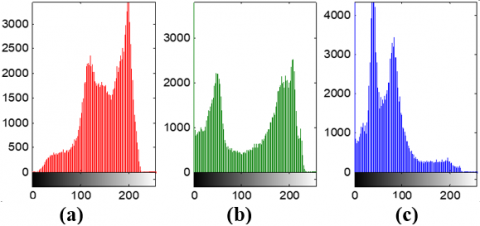Figure 8. Histograms of the three plan color of the original image: (a): Red, (b): Green, (c): Blue

## 9.pngFigure 9. Histograms of the three encrypted plane color of the image: (a): Red, (b): Green, (c): Blue

In order to resist against the statistical attacks, the encrypted image must have histogram with random behavior; the histograms of the original image and the encrypted image are compared in Figures 8, and 9. It is clear that the histograms of the encrypted image are almost uniformly distributed in grayscale [0-255] and don’t provide any indication to statistical attack.

4.1 Correlation coefficients

Table 1 gives the correlation coefficient of the adjacent pixels in all three directions of original and corresponding encrypted images of several test images, we selected randomly 2000 pairs of adjacent pixels in the horizontal, vertical and diagonal directions of the plain images as well as of the encrypted images and we calculated their correlations using the following equations ,

$r_{xy}=|(Cov)|/(√(D(x)×D(y) )$ (2)

$Cov(x,y)=1/N ∑_{i+1}^N(x_i -E(x))(y_i-E(y))$ (3)

$E(x)=1/N ∑_{i=1}^Nx_i$ (4)

$D(x)=1/N ∑_{i=1}^N(x_i-E(x))^2$ (5)

In the above formulas, x and y represent gray values of two adjacent pixels in image, E(x) is the mean, D(x) is the variance and cov(x,y) is the covariance.

From Table 1, it is observed that the encrypted images have significantly lower correlation (very close to zero), which is means that the original images and their encryption are totally different i.e. the encryption images are highly independent to the original images. So our approach is effective against correlation attacks.

Table 1. Correlation coefficients of two adjacent pixels

 Horizontal Vertical Diagonal Original image Encrypted image Original image Encrypted image Original image Encrypted image Peppers.png Red 0.9635 -0.0355 0.9663 -0.0351 0.9564 0.0014 Green 0.9811 -0.0388 0.9818 -0.0346 0.9687 0.0013 Blue 0.9665 -0.0391 0.9664 -0.0366 0.9478 -0.0012 Lena.tif Red 0.9558 -0.0479 0.9781 -0.0540 0.9336 0.0051 Green 0.9401 -0.0615 0.9695 -0.0498 0.9180 0.0084 Blue 0.9189 -0.0471 0.9495 -0.0461 0.8948 0.0108

4.2 Entropies values

Table 2 gives the values of entropy of the original images denoted by E1 and the entropy values of their encryption images E2. The obtained values are very close to the theoretical value 8. This means that information leakage in the encryption process is negligible and the encryption system is secure against the entropy attack. Table 3 gives the entropy values obtained by some existing methods for the grayscale image “lena.tif” of size (256*256). By comparing the results, we observed that the proposed method is better than those obtained in [25-27] and comparable to those obtained in [28, 31].

Table 2. Entropy values

 E1 E2 Peppers.png 7.3388 7.9997 Lena.tif 7.2353 7.9990 E1:entropy value of original image, E2: entropy value of encrypted image.

Table 3. Entropy values of some existing methods

 E2  7.9972  7.9593  7.9968  7.9876  7.9993

4.3 Sensitivity analysis

To evaluate the robustness of image cryptosystems against the key sensitivity, the common measures NPCR (Number of pixels change rate) and UACI (unified average changing intensity) were used. The NPCR measures the percentage number of pixels in difference in two cipher images. It is defined in

$NPCR=(∑_{i,j}D(i,j))/(H×W)×100%$ (6)

Consider two cipher-images, C1 and C2, whose corresponding plain-images have only one pixel difference.

Where D(i, j) is defined as

$D(i,j)=\left\{ \begin{matrix} 0,\text{ }if\text{ }{{c}_{1}}(i,j)={{c}_{2}}(i,j) \\ 1,\text{ }if\text{ }{{c}_{1}}(i,j)\ne {{c}_{2}}(i,j) \\\end{matrix} \right.$ (7)

UACI which is the unified average changing intensity, measures the average intensity of the differences between the plain-image and ciphered image. It is defined by the following formula:

$UACI=1/(H×W)×∑_{i,j}[|C_1 (i,j)-C_2 (i,j)|/255] ×$(8)

The obtained results are given in Table 4, two images are taken for comparison. Higher NPCR values are obtained and the UACI values are in the range of 33 %. The proposed image encryption technique shows extreme sensitivity on the plaintext and hence it is not vulnerable to the differential attacks.

Table 4. Sensitivity parameters

 peppers.png fruit.tif lena.tif flower.tif UACI (%) 32.21 33,46 33,46 33,46 NPCR (%) 99.60 99,60 99,59 99.61

4.5 Key space analysis

The key space should be large enough to make brute force attacks infeasible [29-30]. The proposed algorithm makes use of a key of length 541 bits and therefore an attacker has to try out 2541 (7,198x10162) combinations of the secret key. Table 5 gives a key space comparison of the proposed method with some existing methods in literature; we observed that the key space of the proposed method is enough larger than the existing methods [27, 28, 31]. This demonstrates the security of the proposed system, which needs huge computational resources in order to be violated.

Table 5. Key space of the proposed method compared with some existing methods

 Key Space  2384  2400  2240 Ours 2541

4.6 Speed performance

The execution time of the encryption process is an important factor to considerate for proving the performance of a system. Table 6 gives the time execution of the proposed approach with some test images of different sizes. Table 7 gives comparison of execution time of the proposed method with some existing methods; we take as example an image of size (256*256) pixels. We observed that the execution time is less than the obtained in  and comparable with the obtained in [25, 29].

Table 6. Time execution of the proposed method

 Image size Encryption time(s) Decryption time(s) Lena.tif (256*256) 0.058 0.053 Baboon.bmp (256*256) 0.059 0.054 Peppers.png (512*512*3) 1.08 1.02

Table 7. Performance of the proposed system with 4 existing methods

 Existing methods Encryption time (s) System configuration  0.05261 Matlab R2010a, P-IV, 2.50 GHz, 2 GB RAM.  0.547 ---  0.12 Matlab, AMD Athlon, 2.70GHz, 1GB RAM  0.00297 Matlab7.4,Windows Vista, Pentium Core 2 Duo at 3 GHz. Ours 0.058 Matlab R2010a, Window7, Intel(R) Core(TM)2 DUO CPU@2.93, 2.93GHz, 4GB RAM
5. Conclusions

In this paper we have introduced a new image encryption and decryption scheme. The novelty in this system is the hybridization between the using of the double scanning which is considered as a bloc cipher and the stream cipher (improved achterbahn-128) in order to decrease the executing time and strengthening the security. The experimental results clearly show that our approach provides a good evaluation in terms of visual analysis, statistical analysis and security analysis compared with existing methods. From the execution time calculation, the system is fast and suitable for any kind of color image which can be further extended for video. In the future work, the proposed scheme could be implemented in hardware environments with better security, less computation complexity, less power consumption and small chip area are needed. Finally, further studies should investigate in this domain.

Acknowledgment

We extend our thanks to everyone who helped us in the realization of this work and we also thank LASA laboratory.

References

 Massey JL. (1969). Shift-Register synthesis and BCH decoding. IEEE Transactions on information Theory IT-15: 122-127. http://dx.doi.org/10.1109/TIT.1969.1054260

 Siegenthaler T. (1985). Decrypting a class of stream ciphers using cipher text only. IEEE Transactions on Computers C-34(N1): 81-85.

 Zeng K, Huang M. (1990). On the linear syndrome method in cryptanalysis. In Advance in Cryptology-CRYPTO’88, lecture Notes in Computer Science 405: 469-478. http://dx.doi.org/10.1007/0-387-34799-2_32

 Zeng K, Yang CH, Rao TR. (1991). An improved linear syndrome algorithm in cryptanalysis with applications. In Advance in Cryptology-CRYPTO’90, lecture Notes in Computer Science 537: 34-47. http://dx.doi.org/10.1007/3-540-38424-3_3

 Courtois N, Meier W. (2000). Algebraic Attackson Stream Ciphers with Linear Feedback. Advances in cryptology EUROCRYPT 2003, Lecture Notes in Computer Science 2656: 346-359.

 Mao Y, Chen G. (2003). Handbook of computational geometry for pattern recognition, computer vision, neural computing and robotics. 1-47.

 Belmeguenai A, Berrak O, Mansouri K. (2016). Image encryption using improved keystream generator of achterbahn-128. Proc 11th Joint Conference on Computer Vision, Imaging and Computer Graphics Theory and Applications (VISIGRAPP 2016) 3: 333-339. http://dx.doi.org/10.5220/0005713503330339

 Maniccam SS, Bourbakis NG. (2004). Image and Video encryption using SCAN patterns. Pattern Recognition 37: 725-757. http://dx.doi.org/10.1016/j.patcog.2003.08.011

 Kachris C, Bourbakis N, Dollas A. (2003). A reconfigurable logic based processor for the SCAN image and video encryption algorithm. IJPP 31(6): 489-506. http://dx.doi.org/10.1023/B:IJPP.0000004512.53221.ff

 Saisubha V, Priyanka U, Remya KR, Reenu R. (2013). Image encryption using scan pattern. Proceedings of AECE-IRAJ International Conference.

 Bourbakis NG, Alexopoulos C. (1992). Picutre data encryption using scan pattern. Pattern Recogn 25(6): 567-581. http://dx.doi.org/10.1016/0031-3203(92)90074-s

 Alexopoulos C, Bourbakis NG, Ioannou N. (1995). Image encryption method using a class of fratcals. J. Electron. Imaging 4(3): 251-259. http://dx.doi.org/10.1117/12.208654

 Bourbakis NG. (1997). Image Data Compression -Encryption using G-Scan Pattern. In Proceedings of IEEE International Conference on Systems, Man and Cybernetics 2: 1117-1120. http://dx.doi.org/10.1109/ICSMC.1997.638099

 Chang HKC, Liu JL. (1997). A linear quadtree compression scheme for image encryption. Signal Process. Image Commun. 10(4): 279-290. http://dx.doi.org/10.1016/S0923-5965(96)00025-2

 Chung KL, Chang LC. (1998). Large encryption binary images with higher security. Pattern Recogn. Lett. 19(5-6): 461-468.

 Manniccam SS, Bourbakis NG. (1999). SCAN based lossless image compression and encryption. In Proceeding of IEEE Internaltional Conference on Information Intelligence and Systems (ICIIS’99), pp. 490-499. http://dx.doi.org/10.1109/ICIIS.1999.810321

 Cheng HCH. (1998). Partial encryption for image and video communication. Master’s thesis. University of Alberta.

 Cheng H, Li X. (2000). Partial encryption of compressed images and videos. IEEE Trans signal Process. 48(8): 2439-2451. http://dx.doi.org/10.1109/78.852023

 Chang CC, Yu TX. (2002). Cryptanalysis of an encryption scheme for binary images. Pattern Recogn. Lett. 23(14): 1847-1852. http://dx.doi.org/10.1016/S0167-8655(02)00157-5

 Chen CS, Chen RJ. (2006). Image encryption and decryption using SCAN methodology. Proc. PDCAT.

 Panduranga HT, Kumar SKN. (2010). Hybrid approach for image encryption using SCAN patterns and carrier images. International Journal on Computer Science and Engineering 2(2): 297-300.

 Nagaraju G, HymaLakshmi TV. (2012). Image encryption using secret-key images and scan patterns. Int. J. of Advances in Computer, Electrical & Electronics Eng. 2(S).

 Sivakumar T, Venkatesan R. (2016). A new image encryption method based on knight’s travel path and true random number. J. Of Information Science and Engineering 32: 133-152.

 Stinson DS. (2002). Cryptography: Theory and Practice. Chapman & Hall, New York.

 Loukhaoukha K, Chouinard JY, Berdai A. (2012). A secure image encryption algorithm based on Rubik’s cube principle. Journal of Electrical and Computer Engineering 1-13.

 Ullagaddi V, Hassan F, Devabhaktuni V. (2015). Symmetric synchronous stream encryption using images. SIViP 9(1): 1-8. http://dx.doi.org/10.1007/s11760-012-0416-z

 Hanchinamani G, Kulkarni L. (2015). An efficient image encryption scheme based on a peter de Jong chaotic map and a RC4 stream cipher. 3D Res 6(3): 30-30. http://dx.doi.org/10.1007/s13319-015-0062-7

 Zhang X, Mao Y, Zhao Z. (2014). An efficient chaotic image encryption based on alternate circular S-boxes. Nonlinear Dynamics 78(1): 359-369. http://dx.doi.org/10.1007/s11071-014-1445-7

 Sam S, Devaraj P, Bhuvaneswaran RS. (2012). A novel image cipher based on a mixed transformed logistic maps. Multimedia Tools and Applications 56(2): 315-330. http://dx.doi.org/10.1007/s11042-010-0652-6

 Amin M, Abd El-Latif AA. (2010). Efficient modified RC5 based on chaos adapted to image encryption. J. Electron. Imaging 19(1): 013012. http://dx.doi.org/10.1117/1.3360179

 Chen G, Mao Y, Chui CK. (2004). A symmetric image encryption scheme based on 3D chaotic cat maps. Chaos, Solitions Fractals 21(3): 749-761. http://dx.doi.org/10.1016/j.chaos.2003.12.022

 Huang XL. (2012). Image encryption algorithm using chaotic Chebyshev generator. Nonlinear Dyn 67: 2411-2417.